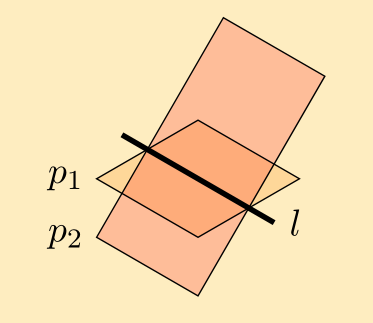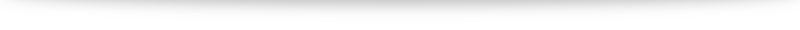# Alternative method to find the line of intersection between two planes

In the video (link to be updated), we went through how to find the line of intersection between two planes using a GC. In this post, we will discuss an alternative method to accomplish this without the use of technology. Just like in our video, we will use the following example:
Find the equation of the line of intersection between $$p_1: \mathrm{r} \cdot \begin{pmatrix} 1 \\ 0 \\ 5 \end{pmatrix} = 7, \quad p_2: \mathrm{r} \cdot \begin{pmatrix} 1 \\ 1 \\ -1 \end{pmatrix} = -3.$$
The two important things we need to find an equation for a line are (1) a point on the line and (2) the direction vector parallel to the line. We will thus tackle the two separately:

#### Finding the direction vector

The key observation here is that the direction vector of the line of intersection is perpendicular to the normal vectors of both planes (try to visualize it!). Thus, to find the direction vector of the line of intersection, we can use the cross product. Hence $$\mathrm{d} = \begin{pmatrix} 1 \\ 0 \\ 5 \end{pmatrix} \times \begin{pmatrix} 1 \\ 1 \\ -1 \end{pmatrix} = \begin{pmatrix} -5 \\ 6 \\ 1 \end{pmatrix}$$

#### Finding a point on the line

To do this, we will first convert the equations of our planes into cartesian form \begin{align} x+5z &=7 \\ x+y-z &= -3 \end{align} Unfortunately, 2 equations with 3 unknowns is not something we commonly encounter or solve. The reason that we have more unknowns than equations is that there are actually many possible points we can find (infinitely many, in fact!). We don't need that many points: we just need one to form our equation of the line. Thus, we can actually specify a value for one of the unknowns and proceed. For example, let $z=0$. Then our equations become \begin{align} x & = 7 \\ x+y&=-3 \end{align} which we can solve to get $x=7$ and $y=-10$, giving us the point $(7,-10,0)$. Letting $z=0$ was just an arbitrary choice, you can actually let one of $x,y$ or $z$ be your favorite number and proceed and you will be able to get a point on the line (different from $(7,-10,0)$, but equally valid nevertheless).

#### Solution

Combining the two parts (point and direction vector) gives us the equation of our line of intersection, $$l: \mathrm{r} = \begin{pmatrix} 7 \\ -10 \\ 0 \end{pmatrix} + \lambda \begin{pmatrix} -5 \\ 6 \\ 1 \end{pmatrix} , \lambda \in \mathbb{R}.$$ You should double check that using your GC will give us the same result (and much faster!)